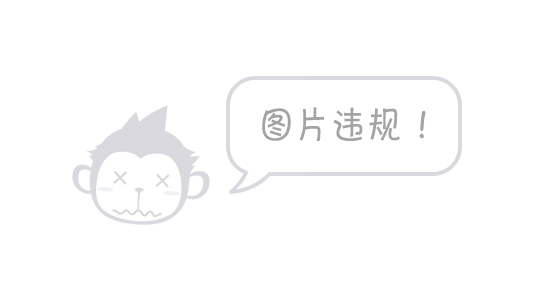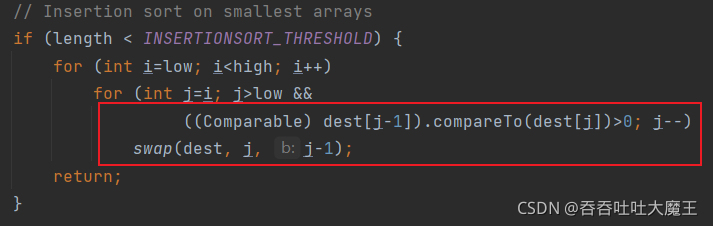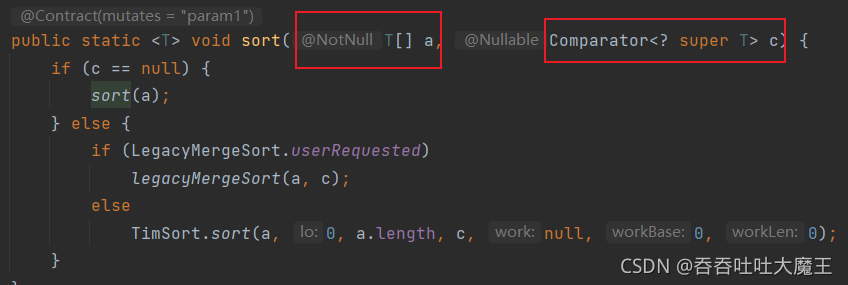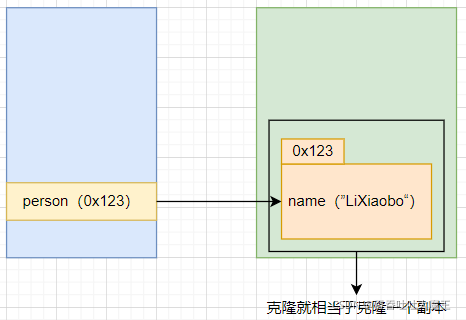【Java 基础语法】万字解析 Java 的多态、抽象类和接口

• 2021 年 11 月 11 日
• 本文字数：8996 字

阅读完需：约 30 分钟

}

}

• 重写时可以修改返回值，方法名和参数类型及个数都不可以修改。仅当返回值为类类型时，重写的方法才可以修改返回值类型，且必须是父类方法返回值的子类；要么就不修改，与父类返回值类型相同

| 区别 | 重载（Overload） | 重写（Override） |

| --- | --- | --- |

| 概念 | 方法名称相同、参数列表不同、返回值无要求 | 方法名称相同、参数列表相同、返回类型一般相同 |

| 范围 | 重载不是必须在一个类当中（继承） | 继承关系 |

| 限制 | 没有权限要求 | 被覆写的方法不能拥有比父类更严格的访问控制权限 |1. 发生向上转型（父类引用需要引用子类对象）

1. 通过父类引用，来调用子类和父类的同名覆盖方法

• 编译的时候： 调用的是父类的方法

• 但是运行的时候： 实际上调用的是子类的方法

4. 向下转型

// 假设 Animal 是父类，Dog 是子类

public class TestDemo{

public static void main(String[] args){

Animal animal=new Animal("动物");

Dog dog=animal;

}

}public class TestDemo{

public static void main(String[] args){

Animal animal=new Animal("动物");

Dog dog=(Dog)animal;

}

}

public class TestDemo{

public static void main(String[] args){

Animal animal=new Animal("动物");

Dog dog=(Dog)animal;

dog.eat();

}

}public class TestDemo{

public static void main(String[] args){

Animal animal=new Dog("二哈");

Dog dog=(Dog)animal;

dog.eat();

}

}

// 假设我的 Dog 类中有一个看家的方法 guard

public class TestDemo{

public static void main(String[] args){

Animal animal=new Dog("二哈");

animal.guard();

}

}

public class TestDemo{

public static void main(String[] args){

Animal animal=new Dog("二哈");

Dog dog =animal;

dog.guard();

}

}

instanceof：可以判定一个引用是否是某个类的实例，如果是则返回 true，不是则返回 false，如

public class TestDemo{

public static void main(String[] args){

Animal animal=new Dog("二哈");

if(animal instanceof Bird){

Bird bird=(Bird)animal;

bird.fly();

}

}

}

5. 关键字 super

| 区别 | this | super |

| --- | --- | --- |

| 概念 | 访问本类中的属性和方法 | 由子类访问父类中的属性和方法 |

| 查找范围 | 先查找本类，如果本类没有就调用父类 | 直接调用父类 |

| 表示 | 表示当前对象 | 无 |

| 共性 1 | 不能被放在 static 修饰的方法中 | 不能被放在 static 修饰的方法中 |

| 共性 2 | 要放在第一行（不能和 super 一起使用） | 要放在第一行（不能和 this 一起使用） |

6. 在构造方法中调用重写方法（坑）

class Animal{

public String name;

public Animal(String name){

eat();

this.name=name;

}

public void eat(){

System.out.println(this.name+"在吃食物（Animal）");

}

}

class Dog extends Animal{

public Dog(String name){

super(name);

}

public void eat(){

System.out.println(this.name+"在吃食物（Dog）");

}

}

public class TestDemo{

public static void main(String[] args){

Dog dog=new Dog("二哈");

}

}• 没有调用 eat 方法，但为什么结果是这样的？

• 为啥是 null？

• 疑惑一： 因为子类继承父类需要帮父类构造方法，所以子类创建对象时，就构造了父类的构造方法，就执行了父类的 eat 方法

• 疑惑二： 由于父类构造方法是先执行 eat 方法，而 name 的赋值在后面一步，多以此时的 name 是 null

7. 理解多态

class Shape{

public void draw(){

}

}

class Cycle extends Shape{

@Override

public void draw() {

System.out.println("画一个圆?");

}

}

class Rect extends Shape{

@Override

public void draw() {

System.out.println("画一个方片?");

}

}

class Flower extends Shape{

@Override

public void draw() {

System.out.println("画一朵花?");

}

}

public class TestDemo{

public static void main(String[] args) {

Cycle shape1=new Cycle();

Rect shape2=new Rect();

Flower shape3=new Flower();

drawMap(shape1);

drawMap(shape2);

drawMap(shape3);

}

public static void drawMap(Shape shape){

shape.draw();

}

}

• 向上转型

• 调用同名的覆盖方法

1）类调用者对类的使用成本进一步降低

• 封装是让类的调用者不需要知道类的实现细节

• 多态能让类的调用者连这个类的类型是什么都不必知道，只需要这个对象具有某种方法即可

2）能够降低代码的“圈复杂度”，避免使用大量的 if-else 语句

public static void drawShapes(){

Rect rect = new Rect();

Cycle cycle = new Cycle();

Flower flower = new Flower();

String[] shapes = {"cycle", "rect", "cycle", "rect", "flower"};

for (String shape : shapes) {

if (shape.equals("cycle")) {

cycle.draw();

} else if (shape.equals("rect")) {

rect.draw();

} else if (shape.equals("flower")) {

flower.draw();

}

}

}

public static void drawShapes() {

// 我们创建了一个 Shape 对象的数组.

Shape[] shapes = {new Cycle(), new Rect(), new Cycle(), new Rect(), new Flower()};

for (Shape shape : shapes) {

shape.draw();

}

}

【一线大厂Java面试题解析+后端开发学习笔记+最新架构讲解视频+实战项目源码讲义】

16#pic_center)

3）可扩展能力强

// 增加三角形

class Triangle extends Shape {

@Override

public void draw() {

System.out.println("△");

}

}

8. 小结1. 概念

class Shape{

public void draw(){

}

}

abstract class Shape{

public abstract void draw();

}

2. 注意事项

• 方法和类都要由 abstract 修饰

• 抽象类中可以定义其他数据成员和成员方法，如

abstract class Shape{

public int a;

public void b(){

// ...

}

public abstract void draw();

}

• 抽象类不可以被实例化

• 抽象方法不能是被 private 修饰的

• 抽象方法不能是被 final 修饰的，它与 abstract 不能被共存

• 如果子类继承了抽象类，但不需要重写父类的抽象方法，则可以将子类用 abstract 修饰，如

abstract class Shape{

public abstract void draw();

}

abstract Color extends Shape{

}

• 一个抽象类 A 可以被另外的抽象类 B 继承，但是如果有其他的普通类继承了抽象类 B，则该普通类需要重写 A 和 B 中的所有抽象方法

3. 抽象类的意义

abstract class Shape{

public int a;

public void b(){

// ...

}

public abstract void draw();

}

class Cycle extends Shape{

@Override

public void draw(){

System.out.println("画一个?");

}

}

public class TestDemo{

public static void main(String[] args){

Shape shape=new Cycle();

}

}

1. 语法规则

interface IShape{

public static void draw();

}

• 接口是使用 interface 定义的

• 接口的命名一般以大写字母 I 开头

• 接口中的方法一定是抽象的、被 public 修饰的方法，因此其中抽象方法可以简化代码为

interface IShape{

void draw();

}

• 接口中也可以包含被 public 修饰的静态常量，并且可以省略 public static final，如

interface IShape{

public static final int a=10;

public static int b=10;

public int c=10;

int d=10;

}

• 接口不能被单独实例化，和抽象类一样需要被子类继承使用，但是接口中使用 implements 继承，如

interface IShape{

public static void draw();

}

class Cycle implements IShape{

@Override

public void draw(){

System.out.println("画一个圆预?");

}

}

• 基础接口的类需要重写接口中的全部抽象方法

• 一个类可以使用 implements 实现多个接口，每个接口之间使用逗号分隔开就可以，如

interface A{

void func1();

}

interface B{

void func2();

}

class C implements A,B{

@Override

public void func1(){

}

@Override

public void func2{

}

}

• 接口和接口之间的关系可以使用 extends 来维护，这是意味着”扩展“，即某个接口扩展了其他接口的功能，如

interface A{

void func1();

}

interface B{

void func2();

}

interface D implements A,B{

@Override

public void func1(){

}

@Override

public void func2{

}

void func3();

}

interface IShape{

void draw();

default public void func(){

System.out.println("默认方法");

}

}

2. 实现多个接口

class Animal{

public String name;

public Animal(String name){

this.name=name;

}

}

class Bird extends Animal{

public Bird(String name){

super(name);

}

}

class Animal{

public String name;

public Animal(String name){

this.name=name;

}

}

interface ISwing{

void swing();

}

interface IFly{

void fly();

}

class Bird extends Animal implements ISwing,IFly{

public Bird(String name){

super(name);

}

@Override

public void swing(){

System.out.println(this.name+"在游");

}

@Override

public void fly(){

System.out.println(this.name+"在飞");

}

}

public class TestDemo {

public static void fly(IFly flying){

flying.fly();

}

public static void main(String[] args) {

IFly iFly=new Bird("飞鸟");

fly(iFly);

}

}

class SuperMan implements IFly{

@Override

public void fly(){

System.out.println("超人在飞");

}

}

public class TestDemo {

public static void fly(IFly flying){

flying.fly();

}

public static void main(String[] args) {

fly(new SuperMan());

}

}

3. 接口的继承4. Comparable 接口

public class TestDemo {

public static void main(String[] args) {

int[] array={2,9,4,1,7};

System.out.println("排序前："+Arrays.toString(array));

Arrays.sort(array);

System.out.println("排序后："+Arrays.toString(array));

}

}

class Student{

private String name;

private int age;

private double score;

public Student(String name, int age, double score) {

this.name = name;

this.age = age;

this.score = score;

}

@Override

public String toString() {

return "Student{" +

"name='" + name + ''' +

", age=" + age +

", score=" + score +

'}';

}

}

public class TestDemo {

public static void main(String[] args) {

Student[] student=new Student;

student=new Student("张三",18,96.5);

student=new Student("李四",19,99.5);

student=new Student("王五",17,92.0);

}

}

public class TestDemo {

public static void main(String[] args) {

Student[] student=new Student;

student=new Student("张三",18,96.5);

student=new Student("李四",19,99.5);

student=new Student("王五",17,92.0);

System.out.println("排序前："+student);

Arrays.sort(student);

System.out.println("排序后："+student);

}

}ClassCastException：类型转换异常，说 Student 不能被转换为 java.lang.Comparableclass Student implements Comparable<Student>{

public String name;

public int age;

public double score;

public Student(String name, int age, double score) {

this.name = name;

this.age = age;

this.score = score;

}

@Override

public String toString() {

return "Student{" +

"name='" + name + '\'' +

", age=" + age +

", score=" + score +

'}';

}

}@Override

public int compareTo(Student o) {

// 新的比较的规则

}@Override

public int compareTo(Student o) {

// 新的比较的规则

return this.age-o.age;

}• 当我们比较上述代码的姓名时，就要将重写的方法改为

@Override

public int compareTo(Student o) {

// 新的比较的规则

return this.name.compareTo(o.name);

}

• 当我们比较上述代码的分数时，就要将重写的方法改为

@Override

public int compareTo(Student o) {

// 新的比较的规则

return int(this.score-o.score);

}

4. Comparator 接口class AgeCompartor implements Comparator<Student>{

}class AgeCompartor implements Comparator<Student>{

@Override

public int compare(Student o1, Student o2) {

return o1.age-o2.age;

}

}

public class TestDemo {

public static void main(String[] args) {

Student[] student=new Student;

student=new Student("张三",18,96.5);

student=new Student("李四",19,99.5);

student=new Student("王五",17,92.0);

System.out.println("排序前："+Arrays.toString(student));

AgeComparator ageComparator=new AgeComparator();

Arrays.sort(student,ageComparator);

System.out.println("排序后："+Arrays.toString(student));

}

}

class NameComparator implements Comparator<Student>{

@Override

public int compare(Student o1, Student o2) {

return o1.name.compareTo(o2.name);

}

}5. Cloneable 接口和深拷贝

class Person{

public String name ="LiXiaobo";

@Override

public String toString() {

return "Person{" +

"name='" + name + ''' +

'}';

}

}

public class TestDemo {

public static void main(String[] args) {

Person person=new Person();

}

}class Person implements Cloneable{

public String name ="LiXiaobo";

@Override

public String toString() {

return "Person{" +

"name='" + name + ''' +

'}';

}

}评论